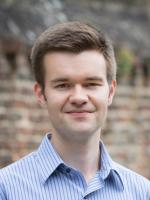# Faculty of MathematicsProfessor of Number Theory

Research Interests:

Number theory and arithmetic geometry

## Publications

$\hat{G}$-local systems on smooth projective curves are potentially automorphic
G Boeckle, M Harris, C Khare, JA Thorne
– Acta Mathematica
(2019)
223,
1
Elliptic curves over $\mathbb{Q}_\infty$ are modular
JA Thorne
– Journal of the European Mathematical Society
(2019)
21,
1943
On the arithmetic of simple singularities of type E
B Romano, JA Thorne
– Research in number theory
(2018)
4,
21
Potential Automorphy and the Leopoldt conjecture
CB Khare, JA Thorne
– American Journal of Mathematics
(2017)
139,
1205
Automorphy of some residually $S_5$ S 5 Galois representations
CB Khare, JA Thorne
– Mathematische Zeitschrift
(2017)
286,
399
A 2-adic automorphy lifting theorem for unitary groups over CM fields
JA Thorne
– Mathematische Zeitschrift
(2017)
285,
1
On the GL$_{n}$-eigenvariety and a conjecture of Venkatesh
D Hansen, JA Thorne
– Selecta Mathematica
(2017)
23,
1205
Level-raising and symmetric power functoriality, III
L Clozel, JA Thorne
– Duke Mathematical Journal
(2017)
166,
325
On the $\phi$-Selmer groups of the elliptic curves y$^2$ = x$^3$ - Dx
DM Kane, JA Thorne
– Mathematical Proceedings of the Cambridge Philosophical Society
(2017)
163,
71
TORSION GALOIS REPRESENTATIONS over CM FIELDS and HECKE ALGEBRAS in the DERIVED CATEGORY
J Newton, JA Thorne
– Forum of Mathematics Sigma
(2016)
4,
e21
• 1 of 3
• >

## Research Group

Number Theory & Algebra

E1.17

01223 337987